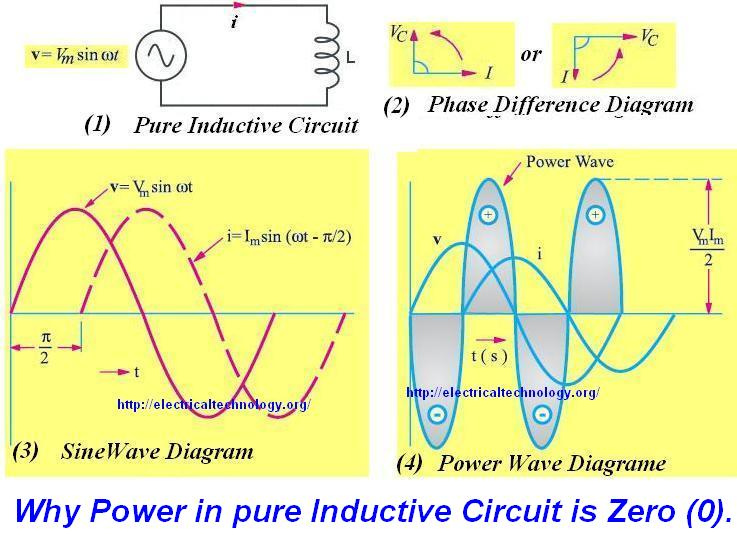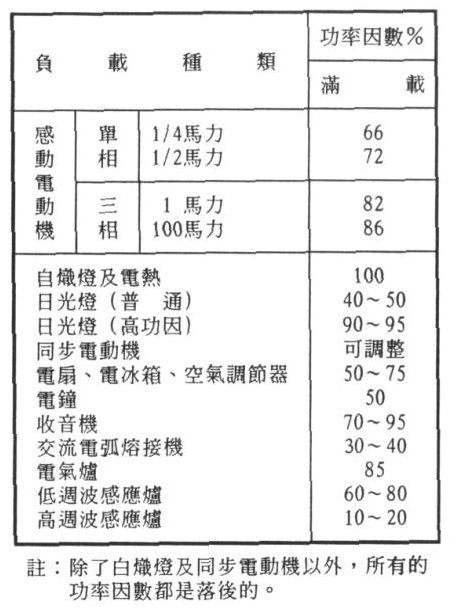## 電功率

2016/8/16 下午 10:20S = VRMS * IRMS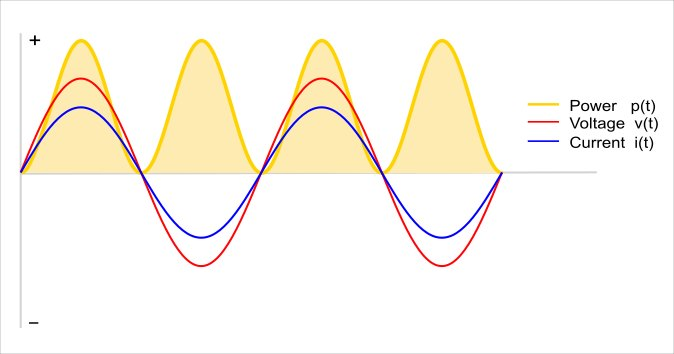S=P+j Q= √((P^2+Q^2 ) ) ∠θ θ=tan^(-1)⁡(Q/P)

pf=P/S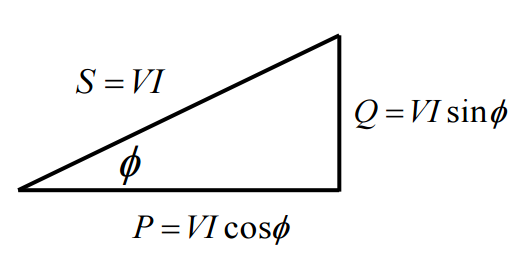pf=P/S= (VI cos⁡θ)/VI= cos⁡〖θ θ=cos^(-1)⁡(pf) 〗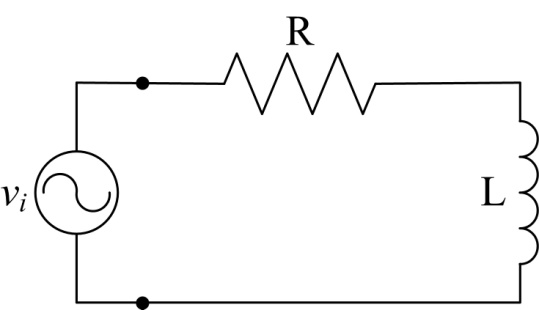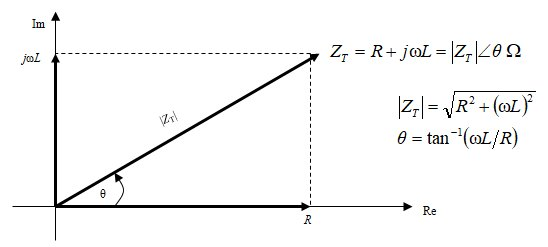Z = 40 + j 75.4 = 85.35 ∠ 62.05o

I = 110 V / 85.35 Ω = 1.29 A

Q = VI * sinθ = 141.9 * sin（62.05o） = 125.32 VAR（同上）